Abstract: Parallel T-type three level inverters have been widely used to expand the rated power of the system. However, when multiple inverters are connected to a single DC bus, the zero sequence circulating current (ZSCC) will be generated. Circulating current inevitably distorts output currents, increases power losses, and reduces the efficiency. This paper introduces the basic working principle of Space Vector Pulse Width Modulation (SVPWM) algorithm in detail, and improves it on the basis of tradition. The simulation is carried out in MATLAB/SIMULINK. The simulation results show that the algorithm has good effect and can lay a good foundation for the actual control system digitization.

1. 引言

2. 逆变器并联系统数学模型

$\left\{\begin{array}{l}VaNx=VLax+{e}_{a}+VoN\\ VbNx=VLbx+{e}_{b}+VoN\\ VcNx=VLcx+{e}_{c}+VoN\end{array}$ (1)

$⇒\left\{\begin{array}{l}{L}_{ax}\cdot \frac{\text{d}{i}_{ax}}{\text{d}t}=VaNx-rx\cdot {i}_{ax}-{e}_{a}-VoN\\ {L}_{bx}\cdot \frac{\text{d}{i}_{bx}}{\text{d}t}=VbNx-rx\cdot {i}_{bx}-{e}_{b}-VoN\\ {L}_{cx}\cdot \frac{\text{d}{i}_{ax}}{\text{d}t}=VcNx-rx\cdot {i}_{cx}-{e}_{c}-VoN\end{array}$ (2)

${i}_{ox}=\frac{1}{3}\underset{k=a,b,c}{\sum }{i}_{kx}$ (3)

${L}_{ox}\cdot \frac{\text{d}{i}_{oz}}{\text{d}t}={V}_{ox}-{r}_{x}\cdot {i}_{oz}-3\cdot VoN$ (4)

$\Delta V={V}_{o1}-{V}_{o2}=\left({L}_{o1}-{L}_{o2}\right)\cdot \frac{\text{d}{i}_{oz}}{\text{d}t}+\left({r}_{1}-{r}_{2}\right)\cdot {i}_{oz}$ (5)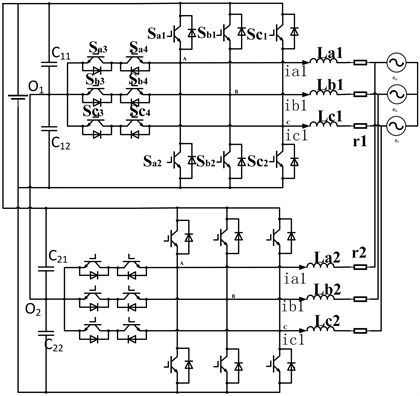Figure 1. Parallel T-type three-level inverter system

3. 基于SVPWM的逆变器并联模型分析

3.1. SVPWM算法原理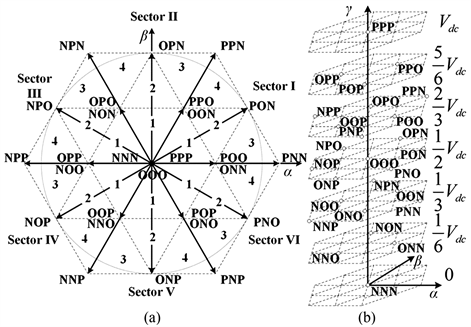Figure 2. (a) Basic space-vector diagram; (b) Zero sequence voltage represented by vector

$Vref=\frac{2}{3}\left(Va+Vb\cdot {\text{e}}^{j\cdot \frac{2\pi }{3}}+Vc\cdot {\text{e}}^{j\cdot \frac{4\pi }{3}}\right)$ (6)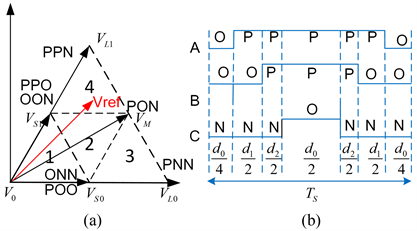Figure 3. (a) Basic space-vector diagram; (b) Zero sequence voltage represented by vector

$\left\{\begin{array}{l}{d}_{0}{V}_{S1}+{d}_{1}{V}_{M}+{d}_{2}{V}_{L1}=Vref\\ {d}_{0}+{d}_{1}+{d}_{2}=1\text{}\end{array}$ (7)

3.2. 由SVPWM引起的环流

$Vo=\frac{Va+Vb+Vc}{6}$ (8)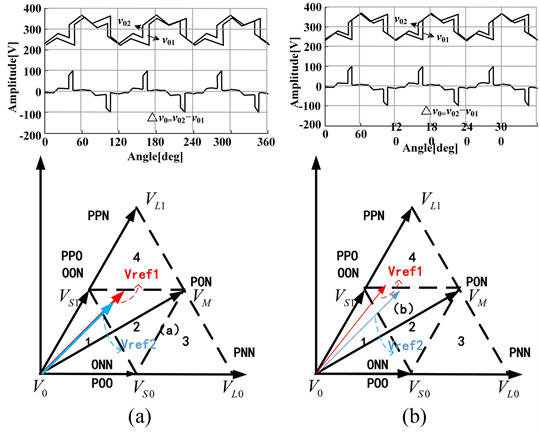Figure 4. (a) Reference vector in phase with different amplitude; (b) Zero sequence voltage represented by vector

${V}_{0}=\frac{{V}_{dc}}{3}\cdot \frac{{d}_{0}}{2}+\frac{{V}_{dc}}{2}\cdot {d}_{1}+\frac{2}{3}\cdot {d}_{2}+\frac{5{V}_{dc}}{6}\cdot \frac{{d}_{0}}{2}=\frac{7{V}_{dc}}{12}\cdot {d}_{0}-\frac{{V}_{dc}}{12}\cdot {d}_{1}+\frac{{V}_{dc}}{12}\cdot {d}_{2}$ (9)

${V}_{0}=\frac{{V}_{dc}}{6}\cdot \frac{{d}_{0}}{2}+\frac{{V}_{dc}}{3}\cdot {d}_{1}+\frac{2}{2}\cdot {d}_{2}+\frac{2{V}_{dc}}{3}\cdot \frac{{d}_{0}}{2}=\frac{5{V}_{dc}}{12}\cdot {d}_{0}-\frac{{V}_{dc}}{12}\cdot {d}_{1}+\frac{{V}_{dc}}{12}\cdot {d}_{2}$ (10)

3.3. NP电压偏差导致的环流

$\left\{\begin{array}{l}e=\left({V}_{c1}-{V}_{c2}\right)/{V}_{dc}\\ -1\le e\le 1\end{array}$ (11)

${V}_{01}=\left(\frac{5}{12}-\frac{{d}_{1}}{12}+\frac{{d}_{2}}{12}+y\right){V}_{dc}+e\left(\frac{-1}{4}-\frac{{d}_{1}}{12}-\frac{{d}_{2}}{4}-\frac{y}{3}\right){V}_{dc}$ (12)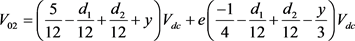(13)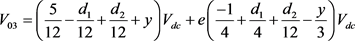(14)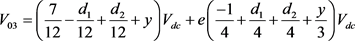(15)

4. 改进后的空间矢量调制算法

4.1. 抑制环流的周期性跳变

1) 将每个扇区划为6个三角形区域，这样幅值差异将不会引起零序电压的周期性跳变。

2) 当有相位差异的并联逆变器的参考矢量落入同一扇区的不同子扇区时，零序电压差值不发生变化，但当参考电压由于相位差异异步地越过两相邻扇区的边界时，零序电压差值会发生跳变，导致环流的跳变。

3) 参考矢量扇区的判断一般是通过其角度和幅值来实现，角度决定了它所落入的大扇区，而通过大扇区的值结合幅值可以判断小扇区。本文通过将并联逆变器的大扇区判断值同步来实现相位差异引起的零序环流的跳变，大扇区同步之后，结合相应的幅值，再进行小扇区的判断，在跨越不同大扇区时候不再引入环流，但这种方法也有缺陷，当并联逆变器参考矢量相位差异过大时该方法将使影响从逆变器的期望输出。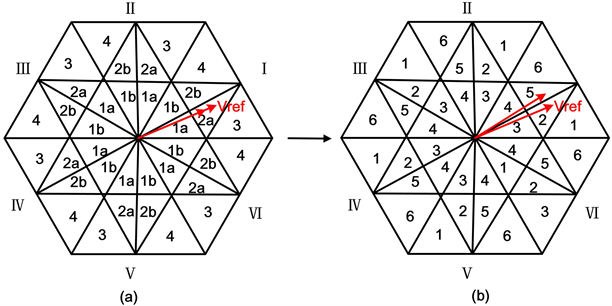Figure 5. (a) Division of six subsectors in each sector for eliminating periodical ZSCC jumps caused by the difference in the amplitudes between reference voltages; (b) New division of sectors for eliminating periodical ZSCC jumps caused by the different phases

4.2. 抑制环流的恒定直流成分Figure 6. Model control block diagram

4.3. 抑制剩余的低频环流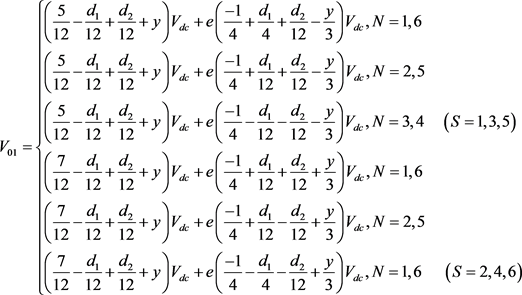(16)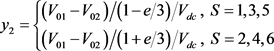(17)

5.1. 矢量控制系统仿真

5.2. 矢量控制系统仿真Figure 7. (a) Inverter output and circulation when amplitude difference is 20 V; (b) Inverter output and circulation when the phase difference is 5˚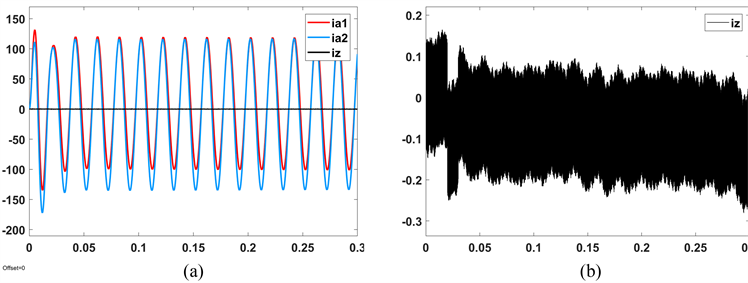Figure 8. (a) Amplitude difference 20 V phase difference 5˚ control effect; (b) Enlarged circulation

6. 结论

 王付胜, 邵章平, 张兴, 等. 多机T型三电平光伏并网逆变器的环流抑制[J]. 中国电机工程学报, 2014, 34(1): 40-49.

 戴明阳, 汪旭东, 许孝卓, 等. 基于Simulink的永磁电机控制系统建模与仿真[J]. 软件导刊, 2018, 17(8): 182-185.

 周胜兰. 基于MATLAB的SPWM电压型逆变器的仿真与分析[J]. 电子技术与软件工程, 2015, 31(6): 38-45.

 Hou, C. (2013) A Multicarrier PWM for Parallel Three Phase Active Frontend Converters. IEEE Transactions on Power Electronics, 59, 290-300.

 Chen, T.P. (2012) Zero-Sequence Circulating Current Reduction Method for Parallel HEPWM Inverters between AC Bus and DC Bus. IEEE Transactions on Industrial Electronics, 59, 290-300.
https://doi.org/10.1109/TIE.2011.2106102

 Mazumder, S.K. (2009) Joint Optimi-zation of Control Performance and Network Resource Utilization in Homogeneous Power Networks. IEEE Transactions on Industrial Electronics, 56, 1736-1745.
https://doi.org/10.1109/TIE.2009.2012458

 周胜兰. 基于MATLAB的SPWM电压型逆变器的仿真与分析[J]. 电子技术与软件工程, 2015, 31(6): 38-45.

 李国丽, 夏秋实, 胡存刚, 等. 三电平NPC逆变器SVPWM方法研究[J]. 电气传动, 2007, 37(12): 31-34.

 邢向洋, 陈阿莲, 张子成, 等. 并联T型三电平逆变器环流抑制和中点平衡研究[J]. 中国电机工程学报, 2016, 36(14): 3909-3919.

Top